Hello, readers welcome to the new tutorial. In this post, we will have a detailed look at Three-Phase Transformation using two Transformers. The transformer is an electric instrument that used to vary the voltage level. In a three-phase transformer, there is three windings are wounded to transform three voltage.

There is wye and delta configuration is used to transformation of voltage. So let’s get started with Three-Phase Transformation using two Transformers,

## Three-Phase Transformation using two Transformers

• For 3 phase transformer connection configuration, there is a technique to do 3 phase transformation with the use of 2 transformers.
• These methods do these operations cause decrement in the power managing ability of transformer but they can be discussed through different conditions.
• The connection arrangement used for 2 transformers is mentioned here.
• The open delta. (or V- V) connection
• The Scott-T connection
• The open-Y -open-.delta. connection
• The three-phase T connection

Let us discuss these connections one by one with the details.

### Open Delta (or V-V) Connection

• In certain conditions, the complete transformer bank is not employed for the construction of three-phase transformation.
• For instance, suppose that delta delta transformer bank consists of the distinct transformer that has faulted phase which should be eliminated for construction.
• The resultant condition can see here.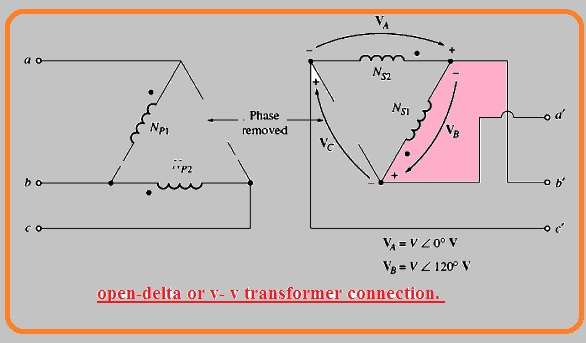• If 2 secondary voltages have values VA = V∠0 and VA = V∠120° Volts so values of voltage about gap here 3rd transformer is employed is mentioned here.• It is a similar voltage that will exist if 3rd transformer is used. Phase C is known as ghost phase. So the open delta configuration caused the transformer bank to obtain through the usage of 2 transformers causing power to move to last when fault eliminated.

How much apparent power can the bank supply with one of its 3 transformers eliminated?

• First of all it looks that it can deliver 2/3 of rated apparent power.Because 2/3 of the transformer is using in the system.
• It does not look a simple state. To observe the result when the transformer is eliminated observe below figure.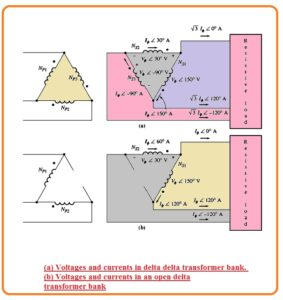• The above diagram denoted by a transformer, bak is operating in normal condition linked with the resistance load
• If the rated voltage of a single transformer in the bank Vθ and rated current is Iθ and highest power given to the load is mentioned here.
• P = 3VθIθcosθ
• The angle between current and voltage is zero
• P = 3VθIθcosθ
• P = 3VθIθ
• The open delta transformer can seen in the above figure denoted as b.
• it is significant to observe that the angle at voltage and current in the transformer bank. Since one transformer phase does not exist the transmission line current has same value to phase current in every transformer and the current and voltage in the transformer bank different in angle through thirty degrees.
• As the current and voltage angle different in every transformer. it is compulsory to observe every transformer on a single basis find the extreme power that can provide to it.
• For transformer one the voltage has an angle of one fifty degrees and the current has an angle of one twenty degrees. Therefore equation for extreme power in the first transformer is mention here.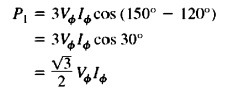• In case of the second transformer, the voltage has an angle of thirty degrees and current at an angle of sixty degrees therefore extreme power is• So the net extreme power of open delta bank is given here.
• P = √3VθIθ
• The rated current has a similar value in every transformer whether that are 3 or 2 and voltage is like at every transformer, therefore, ratio of output power exist through open delta bank to the output power through the general 3 phase bank is mentioned here,• The existing power through open delta bank has a value of 57.7% of real bank value.
• Here question arises
•  What will affect at the other open-delta bank’s rating?
• With that the net power which the 2 transformers can generate is 2/3 of the original bank rating. For finding observe the reactive power of open delta bak.
• The reactive power of first transformer given here.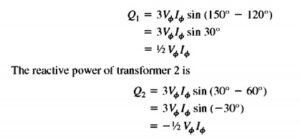• So the transformer is generating reactive power that the other is using. That is energy transformation among the 2 transformer which restricts the power to 57.7% real bank values in place of expected value 66.7%
• The other method to observe the rating of open delta connection is 86.6% of rating the 2 other transformer
• Open delta connections are employed rarely when it is needed to provide less quantity of 3 phase power to single-phase load.
• In this condition, the connection can seen here.• Here transformer denoted as T2 is higher than other

### Open-Wye-Open-Delta Connection

• The open wye open delta connection are like to the open delta connection configuration with the difference that primary voltage are operating through 2 phases and neutral winding.
• This category of connection can see here.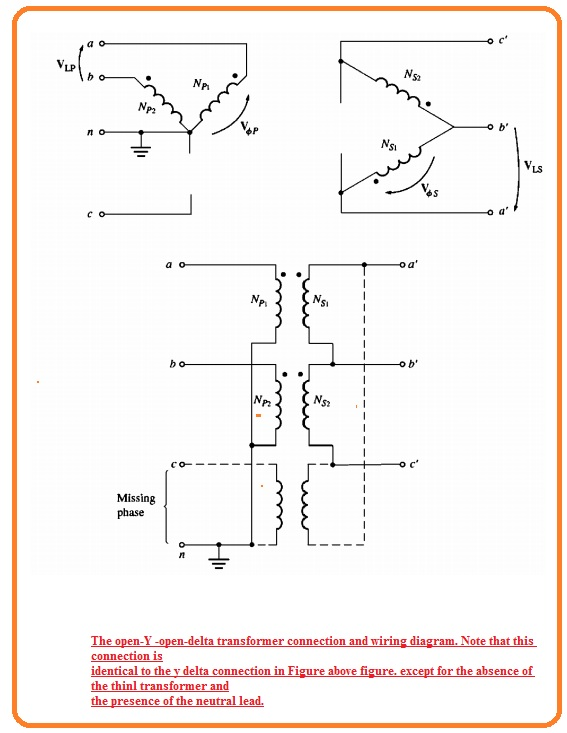• It is operated to run less usage required 3 phase operation in distant places where 3 phase does not exist at power poles.
• For this connection arrangement, the user can obtain 3 phase operation makeshift configuration till the required installation of 3rd phase at power poles.
• The drawback of this arrangement is that high retuning current should pass in the neutral wire of primary circuitry.

### Scott-T Connection Configuration

• The scott T connection is a method to operate 2 phase existing ninety degrees through 3 phase supply.
• In previous times ac supply transmission 2 phases and 3 phases power network mostly used.
• That times it was compulsory to join 2 and 4 phase power network and scott t transformer arrangements was created for this work
• Currently, 2 phase power is used to control operations. butt Scott T is now employed to generate the power required to run that.
• The Scott T comprises of 2 one-phase transformers having same rating values.
• On consists of the tap at its primary side with  86.6 % of full-load voltage. 1
• Can seen in below figure denoted as a• The 86.6 % tap of transformer T2 is linked to the center tap of transformer T1. The voltage given at the primary coil can seen above figure denoted as b, while the resultant voltage given at the primary site of this transformer can be seen in the above figure denoted as c.
• As these voltages are ninety-degree separated the outcomes in 2 phase,

### Three-Phase T Connection

• The Scott T connection are employed 2 transformers to transform 3 phase power to 2 phase power at different voltage values.
• Through a normal variation in the connection, the similar 2 transformers can transform 3 phase power to 3 phase power for values.
• These connections can seen here.• In this configuration, the primary and secondary windings of transformer T1 exist tapped 86.6 % and this tap are linked to the mid tap of resultant coils of transformer T1.
• In this arrangement, T1 is known as the main transformer and T2 teaser transformer
• in the Scott T configuration, 3 phase input voltage generates 2 voltage ninety degrees separated at the primary winding of the transformer.
• This primary voltage generates a secondary voltage that are separated through ninety degrees.
• The main benefit of 3 phase T connection than 3 phase 2 transformer connection is that neutral wire can be linked to primary and secondary sides of the transformer bank
• This connection arrangement is used in self confined 3 phase distribution transformer because its structure price is less than 3 phase transformer bank